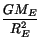Next: Weight and Mass, Revisited Up: Gravity Previous: Newton's Law of Gravity

# Gravitational Acceleration

It is easy to verify that, if air resistence is negligable, all objects accelerate towards the earth at the same rate. This mystery, first verified experimentally by Galileo, is at least partially explained by Newton's law of gravity. The reason'' is that the gravitational force on an object is proportional to its inertial mass. According to Newton's second law, in order to calculate the acceleration of an object caused by gravity, we must take the gravitational force on that object and divide by the inertial mass. Thus, the inertial mass of the object cancels out of the resulting expression for the acceleration. In fact the acceleration of any object at the Earth's surface is determined by the distance of the object form the center of the Earth (RE), Newton's constant (G) and the mass of the Earth:

g =If you put the value of Newton's constant, the radius of the Earth ( 6 x 106 meters) and the mass of the Earth ( 6 x 1024kg) into the above expression you will get approximately 9.8m/s2, which is the rate at which all objects accelerate downwards at the surface of the Earth.

Although the magnitude of the acceleration due to gravity, g, is the same everywhere on the Earth's surface, its direction changes depending on where you are. It is a vector that always points towards the center of the Earth, so, for example, it is in the opposite direction at the North Pole than at the South Pole. This effect is not very relevant to us because the Earth is so big. If we move from one end of the room to another, or even one end of the city to another, we are only moving across a very small fraction of the total circumference of the Earth, so the direction of the gravitational acceleration changes very little. Our notion of down'' only changes significantly when we travel very large distances. However, as we will see later, if you happen to be near a very massive, but small object, such as a black hole, the fact that gravitational acceleration changes direction depending on your location becomes very significant indeed: it gives rise to so-called tidal gravitational forces that can tear a spaceship apart in microseconds.

Note that the rate at which objects accelerates towards the Earth changes with distance. We don't notice this because we are already so far from the center of the Earth (6 thousand kilometers) that we would have to move vertically a large distance to cause a significant change: for example, to decrease the acceleration due to gravity by half down to 4.9 m/s2 we would have to go out into space about 1500 km above the Earth's surface.Next: Weight and Mass, Revisited Up: Gravity Previous: Newton's Law of Gravity
modtech@theory.uwinnipeg.ca
1999-09-29